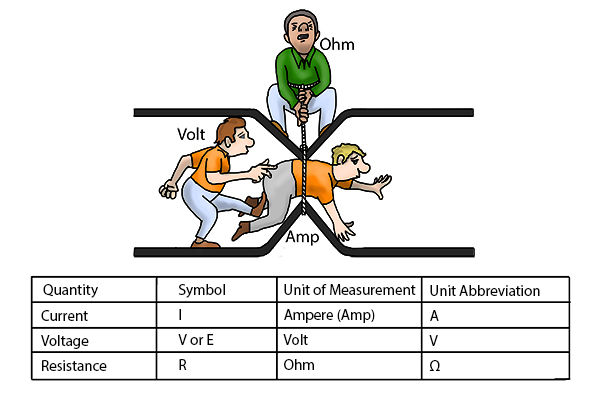top of page
Search

# What is Ohm's law? - Best explanationOhm’s law describes the relationship between the three characteristics of the electrical circuit: the electron flow, the resistance to flow in the wire or other conductor, and the voltage.

The law states that the current through a conductor between two points is directly proportional to the voltage across the two points.Ideally, when a system is functioning properly, it should take 1 volt to push 1 amp thru 1 ohm (Fig.) of resistance. So with Ohm’s law, if you know two of the three parameters in the equation, you can figure out the value of the missing one. For example, if you know the volts and the amps, you can deduce the value of the resistance flowing through the circuit

Sometimes it's hard for students to understand and remember physics laws. However, by showing its practicals and explaining with these kinds of photos students can easily get the concept clarity.

You also might like -

The English Hackathon